Next: Corresponding semi-markovian chain Up: A semi-markovian process Previous: Life expectancy

## Estimating forces from the LSOA survey

At each age, tables such as table 1, provide 4 independent ratios,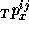, i,j=1,2. If we assume that the 4 unknown forces are constant during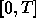, we may estimate these forces. T is the interval between two rounds, i.e. about 2 years.

Matrix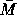from equation 1 can be diagonalized; the eigenvalues are the roots of equation: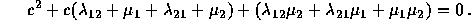Since: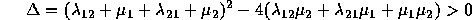, this equation leads to 2 distinct real and negative roots,and, r>s, where: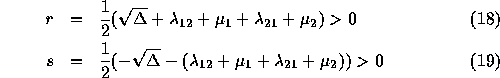Therefore,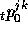, or the probability for an individual in state j at time 0 to be in state k at time t, can be written: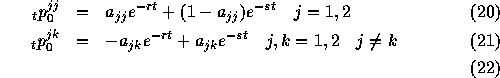Constants are determined by initial conditions at time 0:and by conditions which derivates at time 0 must satisfy: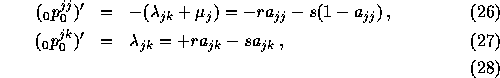Since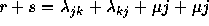, the expression can also be written: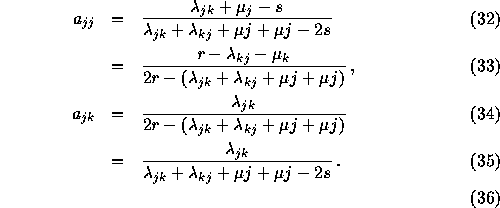If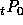corresponds to the (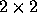) matrix with element, it can be written: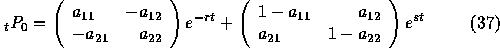As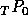is known from the LSOA survey for each single age, this leads to a system of four equations with four unknowns,,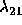,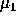and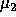.

For the numeric calculations we used IMSL `dneqnf` subroutine. As T is about 2 years, we can either use single age or double age (x, x+2) estimates.

Nicolas Brouard
Tue Jun 6 00:15:46 DF 1995